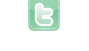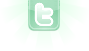# Rosace Curve

```import greenfoot.*;  // (World, Actor, GreenfootImage, Greenfoot and MouseInfo)

/**
* Write a description of class RoseCurve here.
*
* @version (a version number or a date)
*/
public class RoseCurve extends Actor
{
/**
* Act - do whatever the RoseCurve wants to do. This method is called whenever
* the 'Act' or 'Run' button gets pressed in the environment.
*/
double x = 0;
double y = 0;
double newX;
double newY;
int k = 4;

public RoseCurve()
{

}

public void act()
{
drawRoseCurve();

}

public void drawRoseCurve()
{
GreenfootImage gfim = new GreenfootImage(900,600);
gfim.setColor(Color.BLUE);

for (int theta = 0; theta <= 360; theta++) {
double k = theta * Math.PI / 180;
double r = Math.sin(k*theta);

double newX = r*(Math.cos(k));
double newY = r*(Math.sin(k));

gfim.drawLine((int) x, (int) y, (int) newX, (int) newY);

x = newX;
y = newY;
}

setImage(gfim);

}
}
```
I try to make a simple flower with 6 petals I'm trying to understand math Thank you for your help
I would think the radius used would remain constant. Also, when drawing, you should probably start with the center of the image as the "origin".
```public class RosaceCurve extends Actor
{
/**
* Act - do whatever the RoseCurve wants to do. This method is called whenever
* the 'Act' or 'Run' button gets pressed in the environment.
*/
double x1 = 0;
double y1 = 0;
static final int width = 800;
static final int height = 800;
int n = 6;

public void act()
{
drawRosaceCurve();

}

public void drawRosaceCurve()
{
GreenfootImage gfim = new GreenfootImage(width / 2, height / 2);
gfim.setColor(Color.BLUE);

for (int theta = 0; theta <= 360; theta++) {
double k = theta * Math.PI / 180;
double r = 150*(Math.sin(k*n));

double x2 = r*(Math.cos(k));
double y2 = r*(Math.sin(k));

gfim.drawLine((int) x1, (int) y1, (int) x2, (int) y2);

x1 = x2;
y1 = y2;
}

setImage(gfim);

}
}
```
i don't really understand what's wrong the design is 1/4, 3 petals instead of 12 petals
danpost wrote...
when drawing, you should probably start with the center of the image as the "origin".
With center of curve at (0, 0) on image, only the lower-right portion is drawn on image.
thank you I put double x1 = 200 and I add x1 to double x2, it is not the same as if I added 200 to double x2 with double x1 = 0, weird, I thought x1 would be replaced by 200 if I added it to double x2 I don't know if you understood me
ronald wrote...
I put double x1 = 200 and I add x1 to double x2, it is not the same as if I added 200 to double x2 with double x1 = 0, weird, I thought x1 would be replaced by 200 if I added it to double x2
Show revised code.
```public class RosaceCurve extends Actor
{
/**
* Act - do whatever the RoseCurve wants to do. This method is called whenever
* the 'Act' or 'Run' button gets pressed in the environment.
*/
double x1 = 0;
double y1 = 0;
static final int width = 800;
static final int height = 800;
int n = 6;

public void act()
{
drawRosaceCurve();

}

public void drawRosaceCurve()
{
GreenfootImage gfim = new GreenfootImage(width / 2, height / 2);
gfim.setColor(Color.BLUE);

for (int theta = 0; theta <= 360; theta++) {
double k = theta * Math.PI / 180;
double r = 150*(Math.sin(k*n));

double x2 = 200+r*(Math.cos(k));
double y2 = 200+r*(Math.sin(k));

gfim.drawLine((int) x1, (int) y1, (int) x2, (int) y2);

x1 = x2;
y1 = y2;
}

setImage(gfim);

}
}
```
this code works perfectly
```public class RosaceCurve extends Actor
{
/**
* Act - do whatever the RoseCurve wants to do. This method is called whenever
* the 'Act' or 'Run' button gets pressed in the environment.
*/
double x1 = 200;
double y1 = 200;
static final int width = 800;
static final int height = 800;
int n = 6;

public void act()
{
drawRosaceCurve();

}

public void drawRosaceCurve()
{
GreenfootImage gfim = new GreenfootImage(width / 2, height / 2);
gfim.setColor(Color.BLUE);

for (int theta = 0; theta <= 360; theta++) {
double k = theta * Math.PI / 180;
double r = 150*(Math.sin(k*n));

double x2 = x1+r*(Math.cos(k));
double y2 = y1+r*(Math.sin(k));

gfim.drawLine((int) x1, (int) y1, (int) x2, (int) y2);

x1 = x2;
y1 = y2;
}

setImage(gfim);

}
}
```
this code does not work pk ?
ronald wrote...
<< Code Omitted >> this code works perfectly
Good job. However, I would have just change line 32 in your 2nd initial post to:
`gfim.drawLine(200+(int)x1, 200+(int)y1, 200+(int)x2, 200+(int)y2);`
it also works thank you